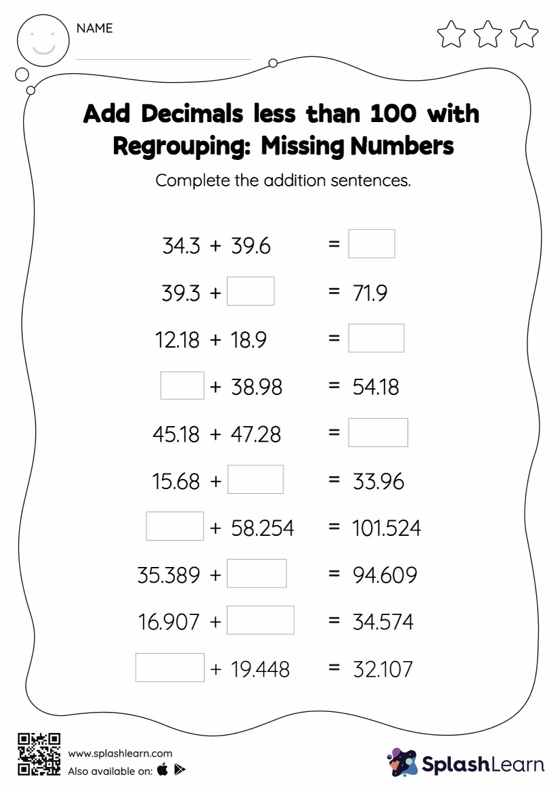# Add Decimals less than 100 with Regrouping: Missing Numbers Worksheet

Home > Add Decimals less than 100 with Regrouping: Missing NumbersAdd decimals less than 100 with regrouping worksheet helps students develop proficiency with addition. Students use the relationship between addition and subtraction to find the missing number while adding decimals. To reach the answer, they must also regroup the numbers in add decimals less than 100 with regrouping worksheet using the relationships between ones, tenths, hundredths, thousandths, etc.